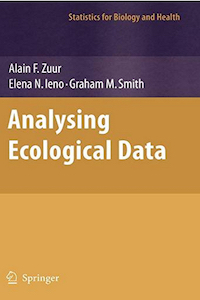www.highstat.com
Highland Statistics Ltd

Latest news

Coming in September 2022:

• Time Series Analysis using Regression Techniques.
• Introduction to GAM and GAMM (frequentist).

See highstat.com for details.# Analyzing Ecological Data (2007). Zuur, Ieno, Smith

This book provides a practical introduction to analyzing ecological data using real data sets collected as part of postgraduate ecological studies or research projects. The first part of the book gives a largely non-mathematical introduction to data exploration, univariate methods (including GAM and mixed modeling techniques), multivariate analysis, time series analysis (e.g. common trends) and spatial statistics.

The first part of the book gives a largely non-mathematical introduction to data exploration, univariate methods (including GAM and mixed modeling techniques), multivariate analysis, time series analysis (e.g. common trends) and spatial statistics. The second part provides 17 case studies, mainly written together with biologists. The case studies include topics ranging from terrestrial ecology to marine biology. The case studies can be used as a template for your own data analysis.

Visit the website of this book to download data, R code, the table of content, and information how to purchase this book.

• Default
• Title
• Date
• Random